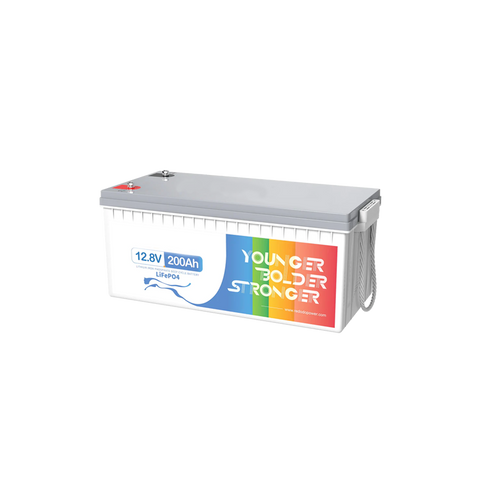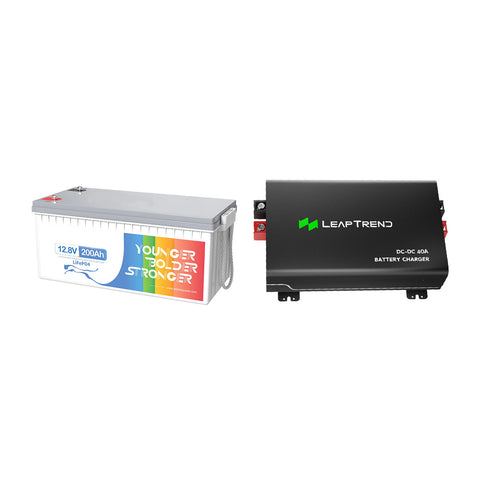# How Many Batteries Are Needed For A 5000 Watt Inverter?

For the power supply requirement of a 5000-watt inverter, you may ask: How many batteries do we need to meet this requirement? In this article, we will explore this question and provide you with a detailed answer.

## Battery capacity for a 5000-watt inverter

When using an inverter for power supply, selecting the right number of batteries is crucial as it determines how long the inverter can provide electricity. For a 5000-watt inverter, we need to consider the following key factors.

Firstly, we need to understand the rated voltage and capacity of the batteries. Common battery voltages are 12 volts or 24 volts, and the capacity is measured in ampere-hours (Ah). For simplification purposes, let's assume we are using 12-volt batteries.Secondly, we need to consider the efficiency of the inverter. Inverters are not 100% efficient, so we need to account for their efficiency factor to ensure the actual output power meets the requirement. Typically, the efficiency of inverters ranges from 90% to 95%.

Now, let's do the calculation. Assuming we have chosen 12-volt batteries and the inverter has an efficiency of 90%. To meet the 5000-watt requirement, we can use the following formula:

Required current (in amperes) = Required power (in watts) / Battery voltage (in volts) / Inverter efficiency

Applying this formula, we calculate the required current:

Required current = 5000 watts / 12 volts / 0.9 (90% efficiency)

The result is approximately 462 amperes.

Therefore, to meet the 5000-watt requirement, you need to choose a battery capacity of at least 462 ampere-hours. Depending on the various battery capacity options available in the market, you can select higher-capacity batteries to extend the power supply time accordingly.

## How much battery capacity does a 2000-watt inverter need?

The size of the battery for an inverter depends on the desired usage time. If we disregard energy losses during inversion, assuming your inverter is 2000 watts with a battery output voltage of 12V, according to the formula P=UI, we can calculate I=P/U and find that the battery output current is 166.67 amperes. Let's suppose your desired usage time is t and the battery capacity is x ampere-hours. In that case, x=166.67*t. However, in practical usage, there will be circuit losses, and as the battery charge decreases, the output voltage U will decrease, resulting in an output current greater than 166.67 amperes. This means that if you want to achieve your desired usage time, the battery capacity provided for the inverter needs to be greater than the result obtained from this calculation.## Battery selection considerations

Firstly, let's discuss the types of batteries. Common battery types include lead-acid batteries, lithium-ion batteries (including lithium iron phosphate batteries), and nickel-metal hydride batteries, each with their advantages and suitable applications. When choosing a battery type, factors such as capacity, cycle life, and price need to be considered.

In terms of battery configuration, series and parallel connections are the key factors to consider. Series connections connect multiple batteries to increase the overall voltage while keeping the battery capacity the same. On the other hand, parallel connections increase battery capacity while maintaining a constant voltage. Choosing the appropriate configuration will provide optimal performance and energy efficiency for your usage.

By understanding the inverter power requirement and the rated voltage and capacity of batteries, you can now better plan the number and capacity of batteries needed to meet the requirements of a 5000-watt inverter.

We hope this article has been helpful in understanding the battery requirements for using a 5000-watt inverter. If you have any further questions, please feel free to contact us, and we will be happy to assist you.